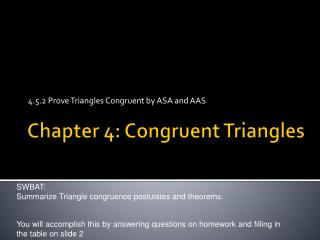DownloadDownload PresentationChapter 4: Congruent Triangles

# Chapter 4: Congruent Triangles

Download Presentation## Chapter 4: Congruent Triangles

- - - - - - - - - - - - - - - - - - - - - - - - - - - E N D - - - - - - - - - - - - - - - - - - - - - - - - - - -
##### Presentation Transcript

1. 4.5.2 Prove Triangles Congruent by ASA and AAS Chapter 4: Congruent Triangles SWBAT: Summarize Triangle congruence postulates and theorems. You will accomplish this by answering questions on homework and filling in the table on slide 2

2. Triangle Congruence Postulates and Theorems • Name the 5 methods we have discussed • Right triangle congruencies: Check P. 252

3. Name the theorem: SAS • Proof: • CB  AD (Given) • BC || AD • ACB  CAD (AIA thm) • CA  AC (Symmetric Property) • Then •  ABC   CDA by SAS B C A D

4. Fill in the necessary information: • Given  CB  CD, ED  EB, CB  ED, ____ _____ • Prove: CBD   EDB by HL E B F A C D

5. Fill in the necessary information: • Given  AB  FD, EF  CA, ____ _____ • Prove: ABC   FDE by SSS E B F A C D

6. Homework • P. 253 • 2, 7, 10, 20, 22, 31, 33